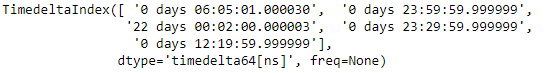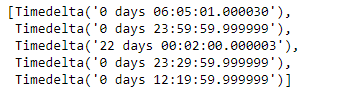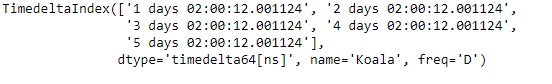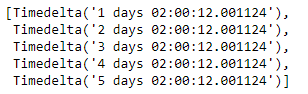Skip to content
Related Articles
Python | Pandas TimedeltaIndex.tolist()
• Last Updated : 06 Jan, 2019

Python is a great language for doing data analysis, primarily because of the fantastic ecosystem of data-centric python packages. Pandas is one of those packages and makes importing and analyzing data much easier.

Pandas` TimedeltaIndex.tolist()` function return a python list of the underlying data in the given TimedeltaIndex object.

Syntax : TimedeltaIndex.tolist()

Parameters : None

Return : list

Example #1: Use `TimedeltaIndex.tolist()` function to return the data of the given TimedeltaIndex object as a python list.

 `# importing pandas as pd``import` `pandas as pd`` ` `# Create the TimedeltaIndex object``tidx ``=` `pd.TimedeltaIndex(data ``=``[``'06:05:01.000030'``, ``'+23:59:59.999999'``,``                        ``'22 day 2 min 3us 10ns'``, ``'+23:29:59.999999'``,``                        ``'+12:19:59.999999'``])`` ` `# Print the TimedeltaIndex object``print``(tidx)`

Output :Now we will use the `TimedeltaIndex.tolist()` function to return the elements of the tidx object as a list.

 `# return a list``tidx.tolist()`

Output :As we can see in the output, the `TimedeltaIndex.tolist()` function has returned a list containing the data of the TimedeltaIndex object.

Example #2: Use `TimedeltaIndex.tolist()` function to return the data of the given TimedeltaIndex object as a python list.

 `# importing pandas as pd``import` `pandas as pd`` ` `# Create the TimedeltaIndex object``tidx ``=` `pd.TimedeltaIndex(start ``=``'1 days 02:00:12.001124'``, ``                        ``periods ``=` `5``, freq ``=``'D'``, name ``=``'Koala'``)`` ` `# Print the TimedeltaIndex object``print``(tidx)`

Output :Now we will use the `TimedeltaIndex.tolist()` function to return the elements of the tidx object as a list.

 `# return a list``tidx.tolist()`

Output :As we can see in the output, the `TimedeltaIndex.tolist()` function has returned a list containing the data of the TimedeltaIndex object.

Attention geek! Strengthen your foundations with the Python Programming Foundation Course and learn the basics.

To begin with, your interview preparations Enhance your Data Structures concepts with the Python DS Course. And to begin with your Machine Learning Journey, join the Machine Learning – Basic Level Course

My Personal Notes arrow_drop_up